### Smooth

Blurs the active image or selection. This filter replaces each pixel with the average of its 3x3 neighborhood.

### Sharpen

Increases contrast and accentuates detail in the image or selection, but may also accentuate noise. This filter uses the following weighting factors to replace each pixel with a weighted average of the 3x3 neighborhood.
```    -1 -1 -1
-1 12 -1
-1 -1 -1
```

### Find Edges

Uses a Sobel edge detector to highlight sharp changes in intensity in the active image or selection. Two 3x3 convolution kernels (show below) are used to generate vertical and horizontal derivatives. The final image is produced by combining the two derivatives using the square root of the sum of the squares.
```     1  2  1     1  0 -1
0  0  0     2  0 -2
-1 -2 -1     1  0 -1
```

### Find Maxima...

Determines the local maxima in an image and creates a binary (mask-like) image of the same size with the maxima, or one segmented particle per maximum, marked. For RGB images, maxima of luminance are selected, with the luminance defined as weighted or unweighted average of the colors depending on the Edit>Options>Conversions settings. This command is based on a plugin contributed by Michael Schmid.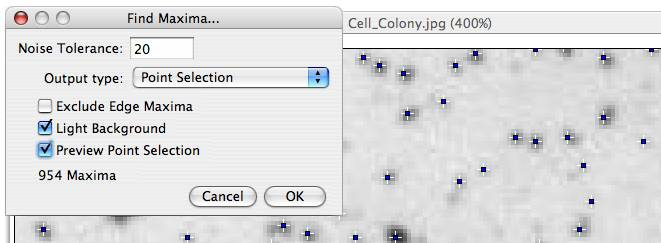A dialog box is displayed with the following options:

• "Noise Tolerance" - Maxima are ignored if they do not stand out from the surroundings by more than this value (calibrated units for calibrated images). In other words, a threshold is set at the maximum value minus noise tolerance and the contiguous area around the maximum above the threshold is analyzed. For accepting a maximum, this area must not contain any point with a value higher at than the maximum. Only one maximum within this area is accepted.
• "Output Type" can be:
• "Single Points" - results in one single point per maximum
• "Maxima Within Tolerance" - all points within the "Noise Tolerance" for each maximum
• "Segmented Particles" - Assumes that each maximum belongs to a particle and segments the image by a watershed algorithm applied to the values of the image (in contrast to Process>Binary>Watershed, which uses the Euclidian distance map).
• "Display Point selection" - Displays a multi-point selection with a point at each maximum
• "Count" - Displays the number of maxima in the Results window
• "Exclude Edge Maxima" - Excludes maxima if the area within the noise tolerance surrounding a maximum touches the edge of the image (edge of the selection does not matter).
• Check Light Background if the image background is brighter than the objects you want to find, as it is in the Cell Colony image in the illustration above.
• "Above Lower Threshold" - (This option appears for thresholded images only.) Finds maxima above the lower threshold only. The upper threshold of the image is ignored. If "Segmented Particles" is selected as "Output Type", the area below the lower threshold is considered a background.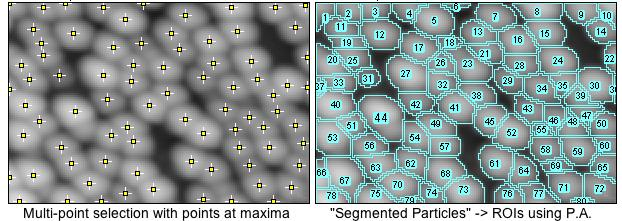Output is a binary image, with foreground 255 and background 0, using an inverted or normal LUT depending on the "Black Background" option in Process>Binary>Options. The number of particles (as obtained by "Analyze Particles") in the output image does not depend on the "Output Type" selected. Note that "Segmented Particles" will usually result in particles touching the edge if "Exclude Edge Maxima" is selected. "Exclude Edge Maxima" applies to the maximum, not to the particle.

Find Maxima does not work on stacks, but the FindStackMaxima macro runs it on all the images in a stack and creates a second stack containing the output images. The FindMaximaRoiManager macro demonstrates how to add particles found by Find Maxima to the ROI Manager.

### Enhance Contrast

Enhances image contrast by using either histogram stretching or histogram equalization. Both methods are described in detail in the Hypermedia Image Processing Reference. Look up "enhancement" in the index.

This command does not alter pixel values as long as the Normalize and Equalize histogram options are not enabled.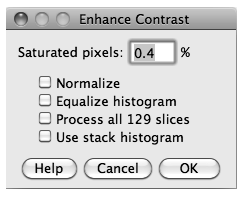Saturated pixels determines the number of pixels in the image that are allowed to become saturated. Increasing this value increases contrast. This value should be greater than zero to prevent a few outlying pixel from causing the histogram stretch to not work as intended.

Check Normalize and ImageJ will recalculate the pixel values of the image so the range is equal to the maximum range for the data type, or 0-1.0 for float images. The maximum range is 0-255 for 8-bit images and 0-65535 for 16-bit images. Note that normalization of RGB images is not supported. The Use stack histogram option is ignored.

With stacks another checkbox, Process all slices, is displayed. If this option is enabled, normalization and histogram equalization are applied to all slices in the stack.

Check Equalize histogram to enhance the image using histogram equalization. Create a selection and the equalization will be based on the histogram of the selection. Uses a modified algorithm that takes the square root of the histogram values. Hold the alt key down to use the standard histogram equalization algorithm. The Saturated pixels and Normalize options are ignored when Equalize Histogram is checked. The equalization code was contributed by Richard Kirk.

If Stack histogram checked, ImageJ will use the overall stack histogram instead of individual slice histograms, that allow optimal adjustments for each slice alone. This option may be especially relevant when performing enhancements based on a ROI.

Use the commands in this submenu to add noise to images or remove it. For more advanced capabilities, check out Erik Meijering's RandomJ package (Binomial, Exponential, Gamma, Gaussian, Poisson and Uniform) at www.imagescience.org/meijering/software/randomj/.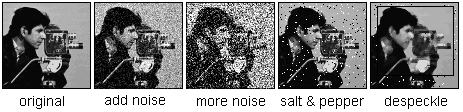Adds random noise to the image or selection. The noise is Gaussian (normally) distributed with a mean of zero and standard deviation of 25.

Adds Gaussian noise with a mean of zero and standard deviation of 75.

### Salt and Pepper

Adds salt and pepper noise to the image or selection by randomly replacing 2.5% of the pixels with black pixels and 2.5% with white pixels. Note: this command only works with 8-bit images.

### Despeckle

This is a median filter. It replaces each pixel with the median value in its 3 x 3 neighborhood. This is a time consuming operation because, for each pixel in the selection, the nine pixels in the 3x3 neighborhood must be sorted and the center pixel replaced with the median value (the fifth). Median filters a good at removing salt and pepper noise.

### Remove Outliers

Replaces a pixel by the median of the pixels in the surrounding if it deviates from the median by more than a certain value (the threshold). Useful for correcting, e.g., hot pixels or dead pixels of a CCD image.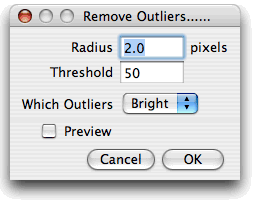Radius determines the area used for calculating the median (uncalibrated, i.e., in pixels). See Process>Filters>Show Circular Masks to see how radius translates into an area. Threshold determinates by how much the pixel must deviate from the median to get replaced, in raw (uncalibrated) units. Which Outliers determines whether pixels brighter or darker than the surrounding (the median) should be replaced.

### Remove NaNs

This filter replaces NaN (Not-a-Number) pixels in 32-bit (float) images by the median of the neighbors inside the kernel area. It does not remove patches of NaNs larger than the kernel size, however.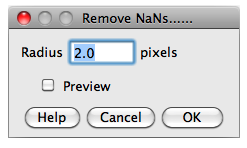Radius determines the area of the circular kernel used for calculating the median. The NaNs macro demonstrates how to create, count and remove NaNs. Note that some ImageJ filters, such as Gaussian Blur, Mean, and Variance destroy the surrounding of NaN pixel by setting it also to NaN. Other filters may produce invalid results in the position of NaN pixels.

Commands in this submenu produce a shadow effect, with light appearing to come from a direction corresponding to the command name. The commands use Convolve3x3, ImageJ's 3x3 convolution function. Two of the convolution kernels are shown in the illustration. Shadows Demo uses all eight kernels to demonstrate the speed of Convolve3x3.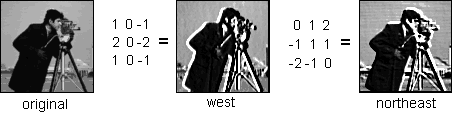This submenu contains commands that create or process binary (black and white) images. They assume that objects are black and background is white unless "Black Background" is checked in the Process>Binary>Options dialog box.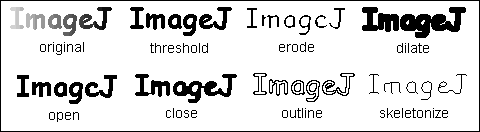### Make Binary

Converts an image to black and white. The threshold level is determined by analysing the histogram of the current selection, or of the entire image if there is no selection. The algorithm used to calculate the threshold is described in the FAQs. If a threshold has been set using the Image>Adjust>Threshold tool, a dialog pops up that lets you specify which pixels are set to the background color and which to the foreground color, and whether the background is black and the foreground is white.

With stacks, all images in the stack are converted to binary using the calculated threshold of the currently displayed slice. Use the ConvertStackToBinary macro to convert a stack to binary using locally calculated thresholds. The MakeSliceBinary macro converts the current stack slice to binary and advances to the next when you press a key.

Converts the image to black and white based on the current threshold settings (if set) or on a threshold calculated by analyzing the histogram. The mask will have an inverting LUT (white is 0 and black is 255) unless "Black Background" is checked in the Process>Binary>Options dialog box.

### Erode

Removes pixels from the edges of black objects. Use Process>Filters>Minimum to do grayscale erosion.

### Dilate

Adds pixels to the edges of black objects. Use Process>Filters>Maximum to do grayscale dilation.

### Open

Performs an erosion operation, followed by dilation. This smoothes objects and removes isolated pixels.

### Close

Performs a dilation operation, followed by erosion. This smoothes objects and fills in small holes.

### Options...

Displays a dialog box that allows several settings used by commands in the Binary submenu to be altered.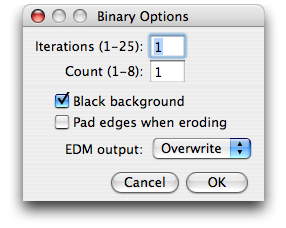Iterations specifies the number of times erosion, dilation, opening, and closing are performed.

Count specifies the number of adjacent background pixels necessary before a pixel is removed from the edge of an object during erosion and the number of adjacent foreground pixels necessary before a pixel is added to the edge of an object during dilation.

Check Black background if the image has white objects on a black background. Plugins can set this option using

```    Prefs.blackBackground = b;
```
and macros can set it using
```    setOption("black background", b);
```
where b is 'true' or 'false'.

If Pad edges when eroding is checked, Process>Binary>Erode does not erode from the edges of the image. This setting also affects Process>Binary>Close, which erodes from the edges unless this checkbox is selected.

EDM output determines the output type for the Process>Binary>Distance Map, Ultimate Points and Voronoi commands. Set it to "Overwrite" for 8-bit output that overwrites the input image; "8-bit", "16-bit" or "32-bit" for separate output images. 32-bit output has floating point (subpixel) distance resolution.

### Outline

Generates a one pixel wide outline of objects in a binary image.

### Skeletonize

Repeatably removes pixels from the edges of objects in a binary image until they are reduced to single pixel wide skeletons.

### Distance Map

Generates a Euclidian distance map (EDM). Each foreground pixel in the binary image is replaced with a gray value equal to that pixel's distance from the nearest background pixel.

### Ultimate Points

Generates the ultimate eroded points (UEPs) of the EDM. Requires a binary image as input. The UEPs represent the centers of particles that would be separated by segmentation. The UEP's gray value is equal to the radius of the inscribed circle of the corresponding particle.

### Watershed

Watershed segmentation is a way of automatically separating or cutting apart particles that touch. It first calculates the Euclidian distance map (EDM) and finds the ultimate eroded points (UEPs). It then dilates each of the UEPs (the peaks or local maxima of the EDM) as far as possible - either until the edge of the particle is reached, or the edge of the region of another (growing) UEP. Watershed segmentation works best for smooth convex objects that don't overlap too much.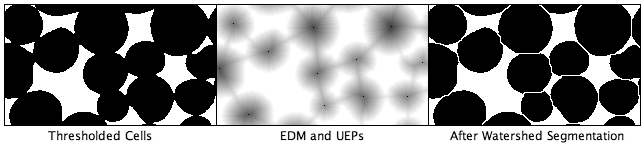Enable debugging in Edit>Options>Misc and the Watershed command will create an animation that shows how the watershed algorithm works. An example is available.

### Voronoi

Splits the image by lines of points having equal distance to the borders of the two nearest particles. Thus, the Voronoi cell of each particle includes all points that are nearer to this particle than any other particle. For the case of the particles being single points, this is a Voronoi tessellation (also known as Dirichlet tessellation).

In the output, the value inside the Voronoi cells is zero; the pixel values of the dividing lines between the cells are equal to the distance to the two nearest particles. This is similar to a medial axis transform of the background, but there are no lines in inner holes of particles. Choose the output type ("Overwrite", "8-bit", "16-bit" or "32-bit") in the Process>Binary>Options dialog box.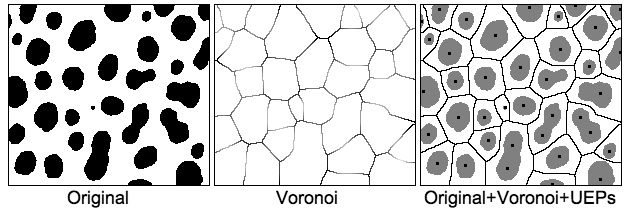The commands in this submenu add (subtract, multiply, etc.) a constant to each pixel in the active image or selection. When the result value overflows/underflows the legal range of the image's data type, the value is reset to the maximum/minimum value. With stacks, a "Process Stack?" dialog is displayed. This dialog has Yes (process entire stack), No (process current image) and Cancel buttons.

Adds a constant to the image or selection. With 8-bit images, results greater than 255 are set to 255. With 16-bit signed images, results greater than 65,535 are set to 65,535.

### Subtract...

Subtracts a constant from the image or selection. With 8-bit and 16-bit images, results less than 0 are set to 0.

### Multiply...

Multiplies the image or selection by the specified real constant. With 8-bit images, results greater than 255 are set to 255. With 16-bit signed images, results greater than 65,535 are set to 65,535.

### Divide...

Divides the image or selection by the specified real constant. Except for 32-bit (float) images, attempts to divide by zero are ignored. With 32-bit images, dividing by zero results in either NaN (0/0) or Infinity.

### AND...

Does a bitwise AND of the image and the specified binary constant.

### OR...

Does a bitwise OR of the image and the specified binary constant.

### XOR...

Does a bitwise XOR of the image and the specified binary constant.

### Min...

Pixels in the image with a value less than the specified constant are replaced by the constant.

### Max...

Pixels in the image with a value greater than the specified constant are replaced by the constant.

### Gamma...

Applies the function f(p) = (p/255)^gamma*255 to each pixel (p) in the image or selection, where 0.1 <= gamma <= 5.0. For RGB images, this function is applied to all three color channels. For 16-bit images, the image min and max are used for scaling instead of 255.

### Set...

Fills the image or selection with the specified value.

### Log...

For 8-bit images, applies the function f(p) = log(p) * 255/log(255) to each pixel (p) in the image or selection. For RGB images, this function is applied to all three color channels. For 16-bit images, the image min and max are used for scaling instead of 255. For float images, no scaling is done. To calculate log10 of the image, multiply the result of this operation by 0.4343 (1/log(10).

### Reciprocal

Generates the reciprocal of the active image or selection. Only works with 32-bit float images.

### NaN Background

Sets non-thresholded pixels in 32-bit float images to the NaN (Not a Number) value. For float images, the "Apply" option in Image>Adjust Threshold runs this command. Pixels with a value of Float.NaN (0f/0f), Float.POSITIVE_INFINITY (1f/0f) or Float.NEGATIVE_INFINITY (-1f/0f) are ignored when making measurements on 32-bit float images.

### Abs

Generates the absolute value of the active image or selection. Only works with 32-bit float images.

### Macro (Expression Evaluator)

This command performs image arithmetic using a user-specified expression. It can be used to create fully-synthetic images or to perform precise pixel manipulations on existing images or stacks. The MathMacroDemo macro, written by Tiago Ferreira, demonstrates how to use it.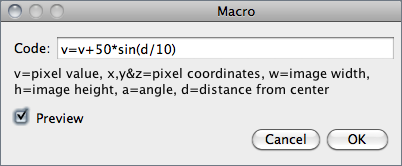The commands in this submenu support frequency domain display, editing and processing. They are based on an implementation of the 2D Fast Hartley Transform (FHT) contributed by Arlo Reeves, the author of the ImageFFT spinoff of NIH Image. For 3D FHTs, check out Bob Dougherty's 3D Fast Hartley Transform plugin.

### FFT

Computes the Fourier transform and displays the power spectrum. The frequency domain image is stored as 32-bit float FHT attached to the 8-bit image that displays the power spectrum. Commands in this submenu, such as Inverse FFT, operate on the 32-bit FHT, not on the 8-bit power spectrum. All other ImageJ commands only "see" the power spectrum.

If the mouse is over an active frequency domain (FFT) window, its location is displayed in polar coordinates. The angle is expressed in degrees, while the radius is expressed in pixels per cycle (p/c). The radius is expressed in [units] per cycle (e.g. mm/c) if the spatial scale of the image was defined using Analyze>Set Scale. With v1.39b or later, the polar coordinates of point selections are recorded by Analyze>Measure. An example is available.

### Inverse FFT

Computes the inverse Fourier transform. You can filter or mask spots on the transformed (frequency domain) image and do an inverse transform to produce an image which only contains the frequencies selected or which suppresses the frequencies selected. Use ImageJ's selection tools and fill/clear commands to draw black or white areas that mask portions of the transformed image. Black areas (pixel value=0) cause the corresponding frequences to be filtered (removed) and white areas (pixel value=255) cause the corresponding frequences to be passed. It is not, however, possible to both filter and pass during the same inverse transform.

Note that areas to be filtered in the frequency domain image must be zero filled and areas to be passed must be filled with 255. You can varify that this is the case by moving the cursor over a filled area and observing that the values displayed in the status bar are either 0 or 255.

This example illustrates how to create masks that remove or pass the low frequencies of an image.With off-center selections, the same spatial frequency appears twice in the power spectrum, at points opposite from the center. With ImageJ 1.41k and later, it is sufficient to fill/clear only one of these. In the following example (courtesy of Arlo Reeves), the cleared selections in the upper half of the power spectrum have been automatically mirrored to the lower half, as shown in the power spectrum of the filtered image.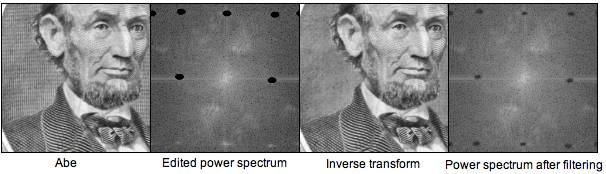The image used in this example is available at rsb.info.nih.gov/ij/images/abe.tif. There is also an example that demonstrates how to remove noise from images generated by a laser scanning confocal microscope.

### Redisplay Power Spectrum

Recomputes the power spectrum from the frequency domain image (32-bit FHT). This command allows you to start over if you mess up while editing the 8-bit power spectrum image.

### FFT Options...

Displays the FFT Options dialog box.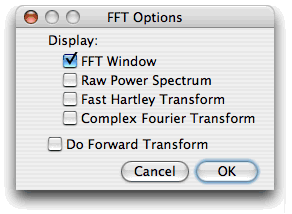• Display - These are checkboxes that specify which image(s) are created by the FFT command:
• FFT Window is the standard output. It consists of an 8-bit image of the power spectrum and the actual data, which remain invisible for the user. The power spectrum image is displayed with logarithmic scaling, enhancing the visibility of components that are weakly visible. The actual data are used for the Inverse FFT command.
• Raw Power Spectrum is the power spectrum without logarithmic scaling.
• Fast Hartley Transform is the internal format used by the command, which is based on a Hartley transform rather than Fourier transform.
• Complex Fourier Transform is a stack with two slices for the real and imaginary parts of the FFT.
• Check Do Forward Transform and the current image is transformed immediately when closing the FFT Options dialog.

### Bandpass Filter..

This is a built in version of Joachim Walter's FFT Filter plugin. It removes high spatial frequencies (blurring the image) and low spatial frequencies (similar to subtracting a blurred image). It can also suppress horizontal or vertical stripes that were created by scanning an image line by line.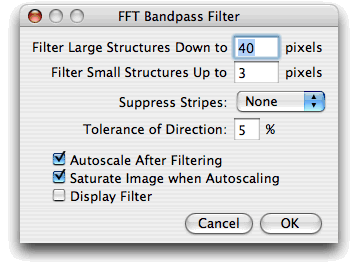• Filter Large Structures Down to - Smooth variations of the image with typical sizes of bright or dark patches larger than this value are suppressed (background).

• Filter Small Structures Up to - Determines the amount of smoothing. Objects in the image smaller than this size are strongly attanuated. Note that these values are both half the spatial frequencies of the actual cutoff. The cutoff is very soft, so the bandpass will noticeably attenuate even spatial frequencies in the center of the bandpass unless the difference of the two values is large (say, more than a factor of 5 or so).

• Suppress Stripes - Select whether to eliminate horizontal or vertical stripes. Removal of horizontal stripes is similar to subtracting an image that is only blurred in the horizontal direction from the original.

• Tolerance of Direction - This is for Suppress Stripes; higher values remove shorter stripes and/or stripes that are running under an angle with respect to the horizontal (vertical) direction.

• Autoscale After Filtering puts the lowest intensity to 0 and the highest intensity to 255, preserving all intensities.

• Saturate allows some intensities to go into saturation, and produces a better visual contrast. Saturate only has an effect when Autoscale is enabled.

• Display Filter shows the filter generated. Note that this disables Undo of the filter operation on the original image.
The Bandpass Filter uses a special algorithm to reduce edge artifacts (before the Fourier transform, the image is extended in size by attaching mirrored copies of image parts outside the original image, thus no jumps occur at the edges).

### Custom Filter..

This command does fourier space filtering of the active using a user-supplied image as the filter. This command does Fourier space filtering of the active image using a user-supplied image as the filter. This image will be converted to 8-bits. For pixels that have a value of 0, the corresponding spatial frequences will be blocked. Pixel with values of 255 should be used for passing the respective spatial frequencies without attenuation. Note that the filter should be symmetric with respect to inversion of the center: Points that are opposite of the center point (defined as x=width/2, y=height/2) should have the same value. Otherwise, artifacts can occur.

For some examples, see the FFTCustomFilterDemo and FFTRemoveStreaks macros.

### FD Math..

This command correlates, convolves or deconvolves two images. It does this by converting the images to the frequency domain, performing conjugate multiplication, multiplication or division, then converting the result back to the space domain. These three operations in the frequency domain are equivalent to correlation, convolution and deconvolution in the space domain. Refer to the DeconvolutionDemo and MotionBlurRemoval macros for an examples.

This submenu contains miscellaneous filters and plugin filters that have been installed by the Plugins>Utilities>Install Plugin command. For more information, refer to the Hypermedia Image Processing Reference at http://www.dai.ed.ac.uk/HIPR2/. Click on Index and look up the keywords convolution, Gaussian, median, mean, erode, dilate and unsharp.

### Convolve...

Does spatial convolution using a kernel entered into a text area. A kernel is a matrix whose center corresponds to the source pixel and the other elements correspond to neighboring pixels. The destination pixel is calculated by multiplying each source pixel by its corresponding kernel coefficient and adding the results. If needed, the input image is effectively extended by duplicating edge pixels outward. There is no arbitrary limit to the size of the kernel but it must be square and have an odd width.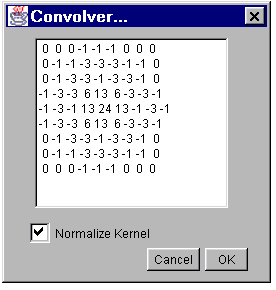Rows in the text area must all have the same number of coefficients, the rows must be terminated with a carriage return, and the coefficients must be separated by one or more spaces. Kernels can be pasted into the text area using the ctrl+v keyboard shortcut. Checking Normalize Kernel causes each coefficient to be divided by the sum of the coefficients, preserving image brightness.

The kernel shown is a 9 x 9 "Mexican hat", which does both smoothing and edge detection in one operation. Note that kernels can be saved as a text file by clicking on the "Save" button, displayed as an image using File>Import>Text Image, scaled to a reasonable size using Image>Adjust>Size and plotted using Analyze>Surface Plot.

The ConvolutionDemo macro demonstrates how to use this command in a macro.

### Gaussian Blur...

This filter uses convolution with a Gaussian function for smoothing. Sigma is the radius of decay to exp(-0.5) ~ 61%, i.e. the standard deviation sigma of the Gaussian (this is the same as in Photoshop, but different from earlier versions of ImageJ, where a value 2.5 times as much had to be entered.

Like all ImageJ convolution operations, it assumes that out-of-image pixels have a value equal to the nearest edge pixel. This gives higher weight to edge pixels than pixels inside the image, and higher weight to corner pixels than non-corner pixels at the edge. Thus, when smoothing with very high blur radius, the output will be dominated by the edge pixels and especially the corner pixels (in the extreme case, with a blur radius of e.g. 1e20, the image will be raplaced by the average of the four corner pixels).

For increased speed, except for small blur radii, the lines (rows or columns of the image) are downscaled before convolution and upscaled to their original length thereafter.

### Median...

Reduces noise in the active image by replacing each pixel with the median of the neighboring pixel values.

### Mean...

Smooths the current image by replacing each pixel with the neighborhood mean. The size of the neighborhood is specified by entering its radius in a dialog box.

### Minimum...

This filter does grayscale erosion by replacing each pixel in the image with the smallest pixel value in that pixel's neighborhood.

### Maximum...

This filter does grayscale dilation by replacing each pixel in the image with the largest pixel value in that pixel's neighborhood.

Unsharp masking subtracts a blurred copy of the image and rescales the image to obtain the same contrast of large (low-frequency) structures as in the input image. This is equivalent to adding a high-pass filtered image and thus sharpens the image. Radius is the standard deviation (blur radius) of the Gaussian blur that is subtracted. Mask Weight determines the strength of filtering, whereby Mask Weight=1 would be an infinite weight of the high-pass filtered image that is added.

### Variance...

Heighlights edges in the image by replacing each pixel with the neighborhood variance.

Generates a stack containing examples of the circular masks used by the Median, Mean, Minimum, Maximum and Variance filters for various neighborhood sizes.

### Image Calculator...

Performs arithmetic and logical operations between two images selected from popup menus. Image1 or both Image1 and Image2 can be stacks. If both are stacks, they must have the same number of slices. Image1 and Image2 must be the same data type but they do not have to be the same size.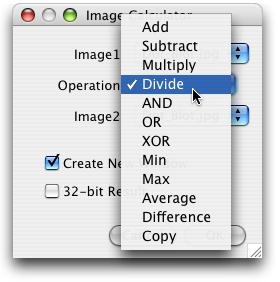You can select one of 12 operators from the Operation: popup menu. Check Create New Window and a new image or stack will be created to hold the result. Otherwise, the result of the operation replaces some or all of Image1. Check "32-bit Result" and the source images will be converted to 32-bit floating point before the specified operation is performed.

With 32-bit (float) images, pixels resulting from division by zero are set to Infinity, or to NaN (Not a Number) if a zero pixel is divided by zero. The divide-by-zero value can be redefined in Edit>Options>Misc.

 Add img1 = img1+img2 Subtract img1 = img1-img2 Multiply img1 = img1*img2 Divide img1 = img1/img2 AND img1= img1 AND img2 OR img1 = img1 OR img2 XOR img1 = img1 XOR img2 Min img1 = min(img1,img2) Max img1 = max(img1,img2) Average img1 = (img1+img2)/2 Difference img1 = |img1-img2| Copy img1 = img2

In these examples, the source and destination have inverted LUTs so zero pixels are white. Operations on images with non-inverted LUTs, and RGB images, will not produce the same results.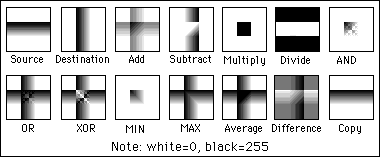### Subtract Background...

Removes smooth continuous backgrounds from gels and other images. Based on the "rolling ball" algorithm described in Stanley Sternberg's article, "Biomedical Image Processing", IEEE Computer, January 1983. Imagine a 3D surface with the pixel values of the image being the height, then a ball rolling over the back side of the surface creates the background. The current algorithm (since v1.39f) uses an approximation of a paraboloid of rotation instead of a ball.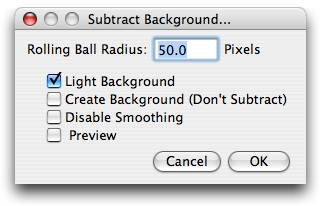The Rolling Ball Radius is the radius of curvature of the paraboloid. As a rule of thumb, for 8-bit or RGB images it should be at least as large as the radius of the largest object in the image that is not part of the background. Larger values will also work unless the background of the image is too uneven. For 16-bit and 32-bit images with pixel value ranges different from 0-255, the radius should be inversely proportional to the pixel value range. For example, typical values of the radius are around 0.2 to 5 for 16-bit images (pixel values 0-65535).

The Light Background option allows the processing of images with bright background and dark objects.

With the Create Background option, the output is not the image with the background subtracted but rather the background itself. This option is useful for examining the background created (in conjunction with the Preview option). Create Background can be also used for custom background subtraction algorithms where the image is duplicated and filtered (e.g. removing "holes" in the background) before creating the background and finally subtracting it with Process>Image Calculator.

For calculating the background ("rolling the ball"), images are maximum-filtered (3x3 pixels) to remove outliers such as dust and then smoothed to reduce noise (average over 3x3 pixels). With Disable Smoothing, the unmodified image data are used for creating the background. Check this option to make sure that the image data after subtraction will never be below the background.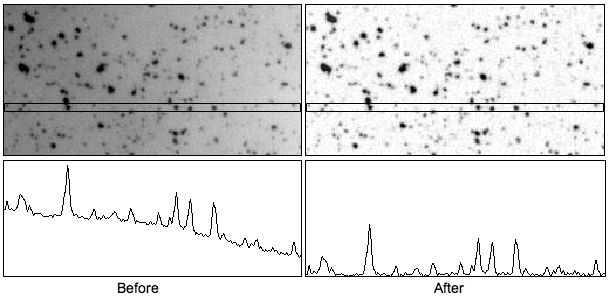### Repeat Command

Reruns the previous command. The Undo and Open commands are skipped. For a shortcut, type shift-R.